# R语言分析负利率下金融市场：负利率和年金价值的变化

### 隐马尔科夫模型hmm在股市中的应用

20203月 –弄清楚何时开始或何时止损，调整风险和资金管理技巧，都取决于股市的当前状况。

``````> PRIX=function(annee=2011,age,sex="HOM",
+ taux=0.04, duree,C=1000){

+  an    = annee-age; if(an>2005){an=2005}
+  nom   = paste("X",an,sep="")
+  L     = B[,nom]
+  Q     = L[(age+1):length(L)]/L[(age+1)]
+  actualisation = (1+taux)^(0:min(duree,120-age))
+  prixsup = sum(Q[2:(min(duree,120-age)+1)]/
+  actualisation[2:(min(duree,120-age)+1)] )
+   prixinf = sum(Q[1:(min(duree,120-age))]/
+  actualisation[1:(min(duree,120-age))] )
+  return(C*c(prixsup,prixinf))}``````

​我在这里指两个年金“价格”，具体取决于年金是在年末还是年初支付的。

``````> PRIX(age=50,sex="HOM",duree=150,taux=.05)
 16435.31 17435.30``````

``````> PRIX(age=50,sex="HOM",duree=150,taux=0)
 38822.48 39822.46``````

``````
> plot(vage,vpn2,type="b",col="red")
> lines(vage,vp0,type="b")
> lines(vage,vp2,type="b",col="blue")
> legend("topright",c("taux -2%","taux 0%","taux 2%"),col=c("red","black","blue"))``````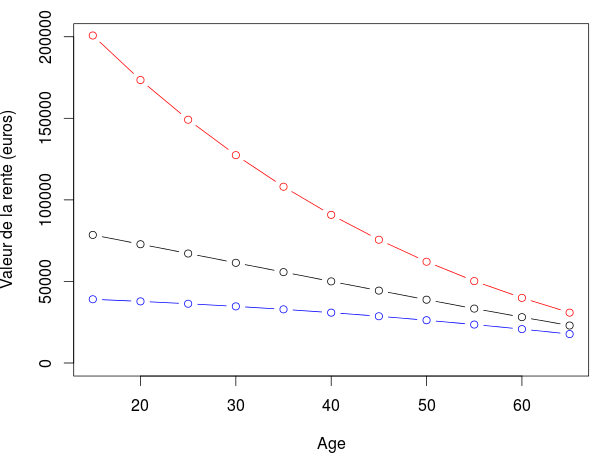``````> PRIX(age=15,sex="HOM",duree=150,taux=.05)
 19427.94 20427.93
> PRIX(age=15,sex="HOM",duree=150,taux=-.02)
 200778.3 201770.3``````

``````> plot(vage,vpn2/vp5*100,type="b",col="red")
> lines(vage,vp0/vp5*100,type="b")
> lines(vage,vp2/vp5*100,type="b",col="blue")
> abline(h=100,lty=2)
> legend("topright",c("taux -2%","taux 0%","taux 2%"),col=c("red","black","blue"),pch=1)``````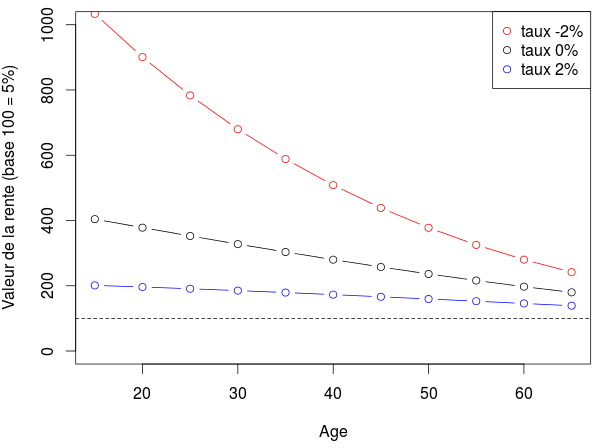# 年金利率和价值的演变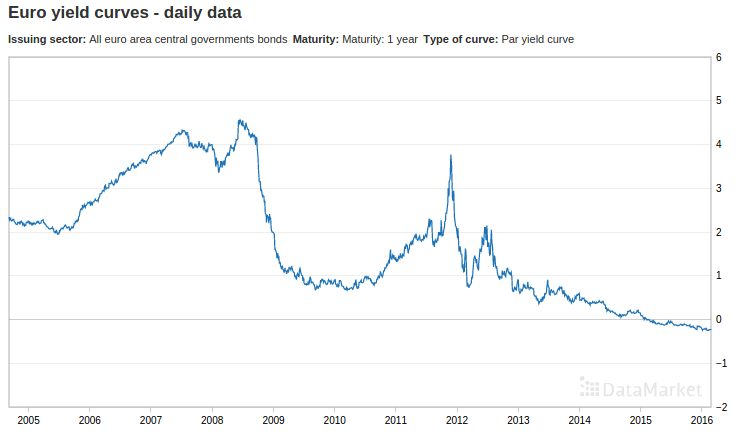``````
> plot(D,Y,type="l")
> abline(h=0,col="red")``````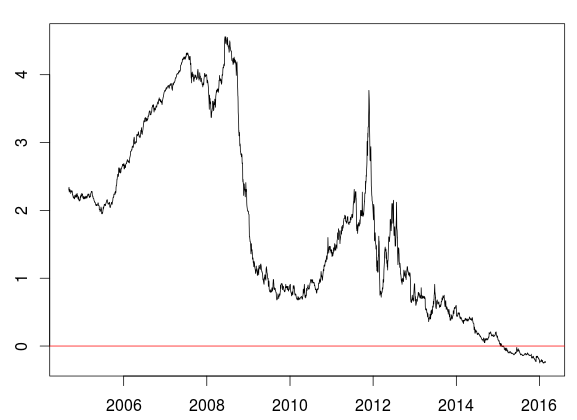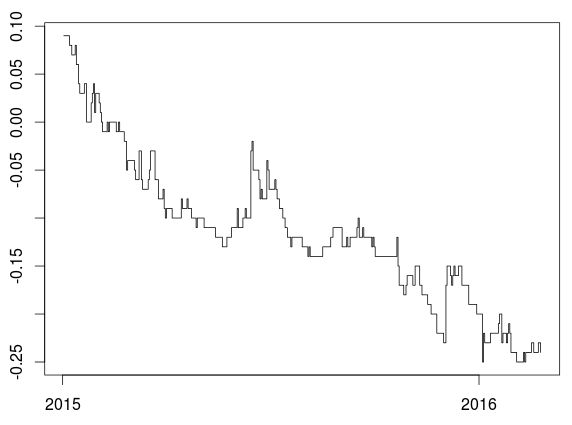``> rente15H=Vectorize(F15)(seq(1,length(Y),by=1))``

``> rente15F=Vectorize(F15)(seq(1,length(Y),by=1))``

``> rente25H=Vectorize(F25)(seq(1,length(Y),by=1))``

``> rente25F=Vectorize(F25)(seq(1,length(Y),by=1))``

``````> plot(D,b15f*100,col="red",type="l")
> lines(D,b15h*100,col="blue")``````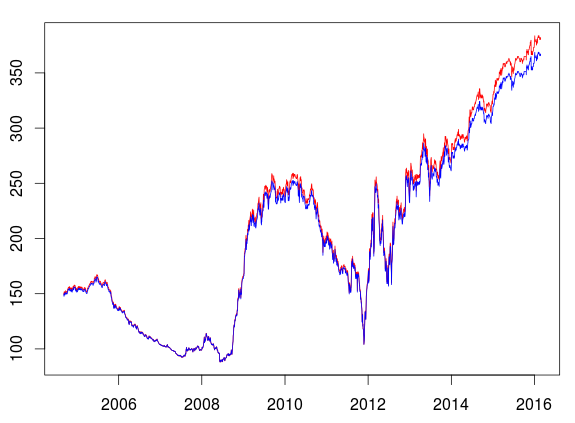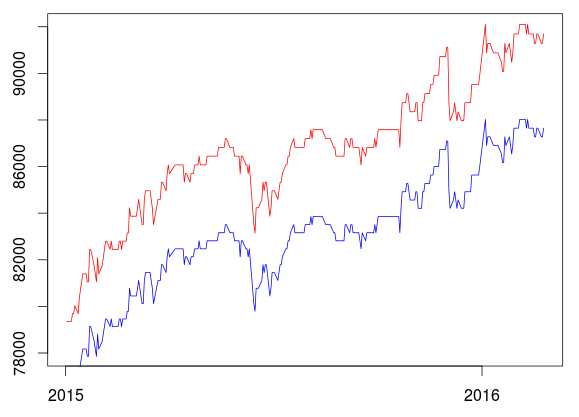``````> plot(D,b25f*100,col="red",type="l")
> lines(D,b25h*100,col="blue")``````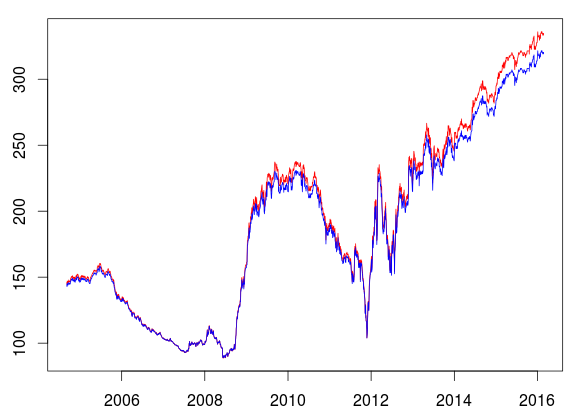Kaizong Ye拓端研究室（TRL）的研究员。

​非常感谢您阅读本文，如需帮助请联系我们！QQ在线咨询

15121130882

0571-63341498

## 关注有关新文章的微信公众号

This will close in 0 seconds